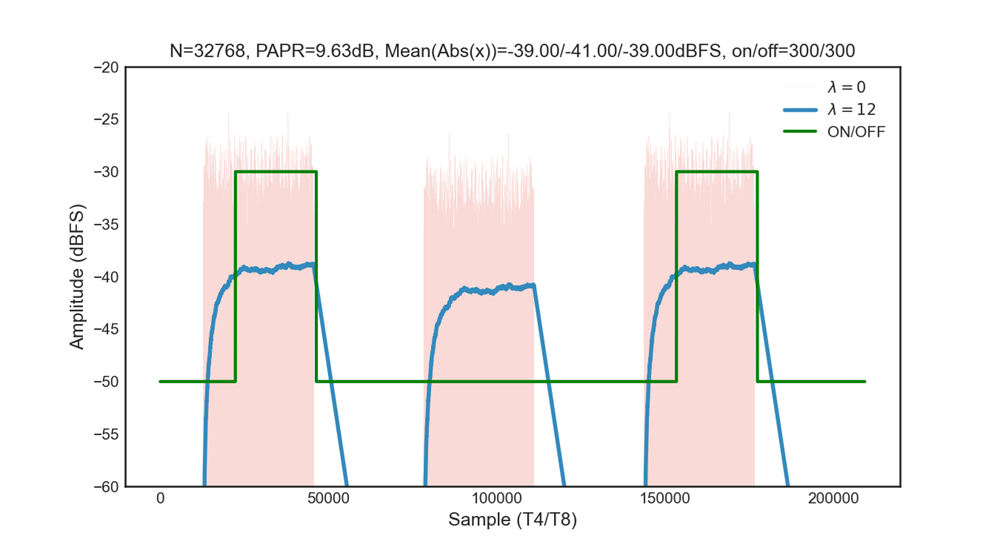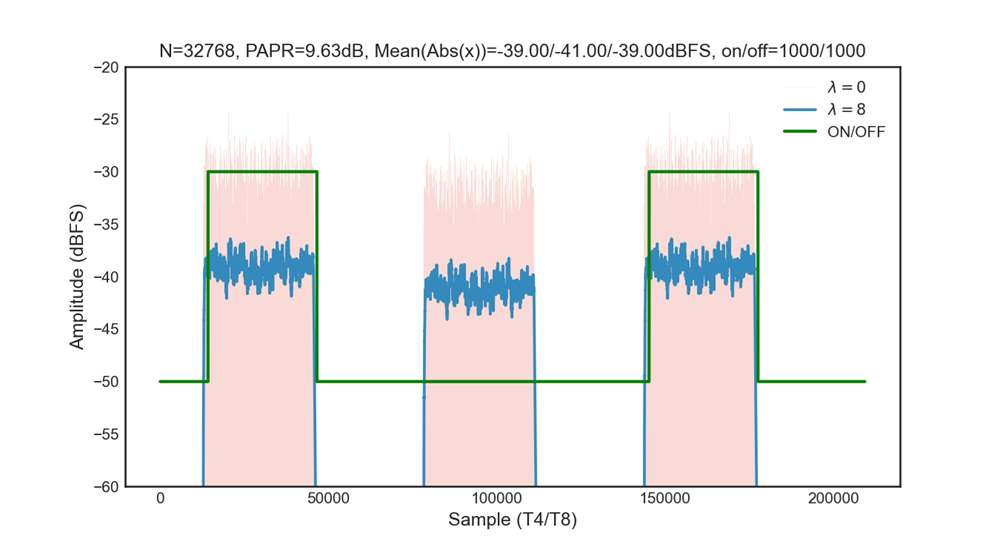# Signal Detector Examples - 2.6 English

## Zynq UltraScale+ RFSoC RF Data Converter Gen 1/2/3/DFE LogiCORE IP Product Guide (PG269)

Document ID
PG269
Release Date
2022-10-21
Version
2.6 English

With the threshold setting to -40 dBFS, the following examples illustrate the response of this signal detector module with different time constants and counter values.

Assuming the sampling rate is 4 GHz, with interleaving factor of 8, each T8 is 2 ns.

Assuming the on and off counter values are 1000 for lambda = 8 and 300 for lambda = 12, which give the rising time of (587 or 9429 + 2*1000 or 2*300) * T8, around 5.2 µs or 20 µs; The falling time should be considered falling from -39 dBFS to -41 dBFS in this example, which is around (58 or 942 + 2*1000 or 2*300) * T8, hence 4.1 µs or 3.1 µs.

The following figures show two examples, both based on high PAR (around 12 dB) burst signal and the signals amplitude are around -40 dBFS with a little bit offset (1-2 dB).

Figure 1. Response with lambda = 12The above figure illustrates the response with lambda = 12 and counter = 300.

Figure 2. Response with lambda = 8The above figure illustrates the response with lambda = 8 and counter = 1000.

These examples show proper response are given from this module base on the input signal amplitude. You should choose counter values and a proper time constant for specific applications.